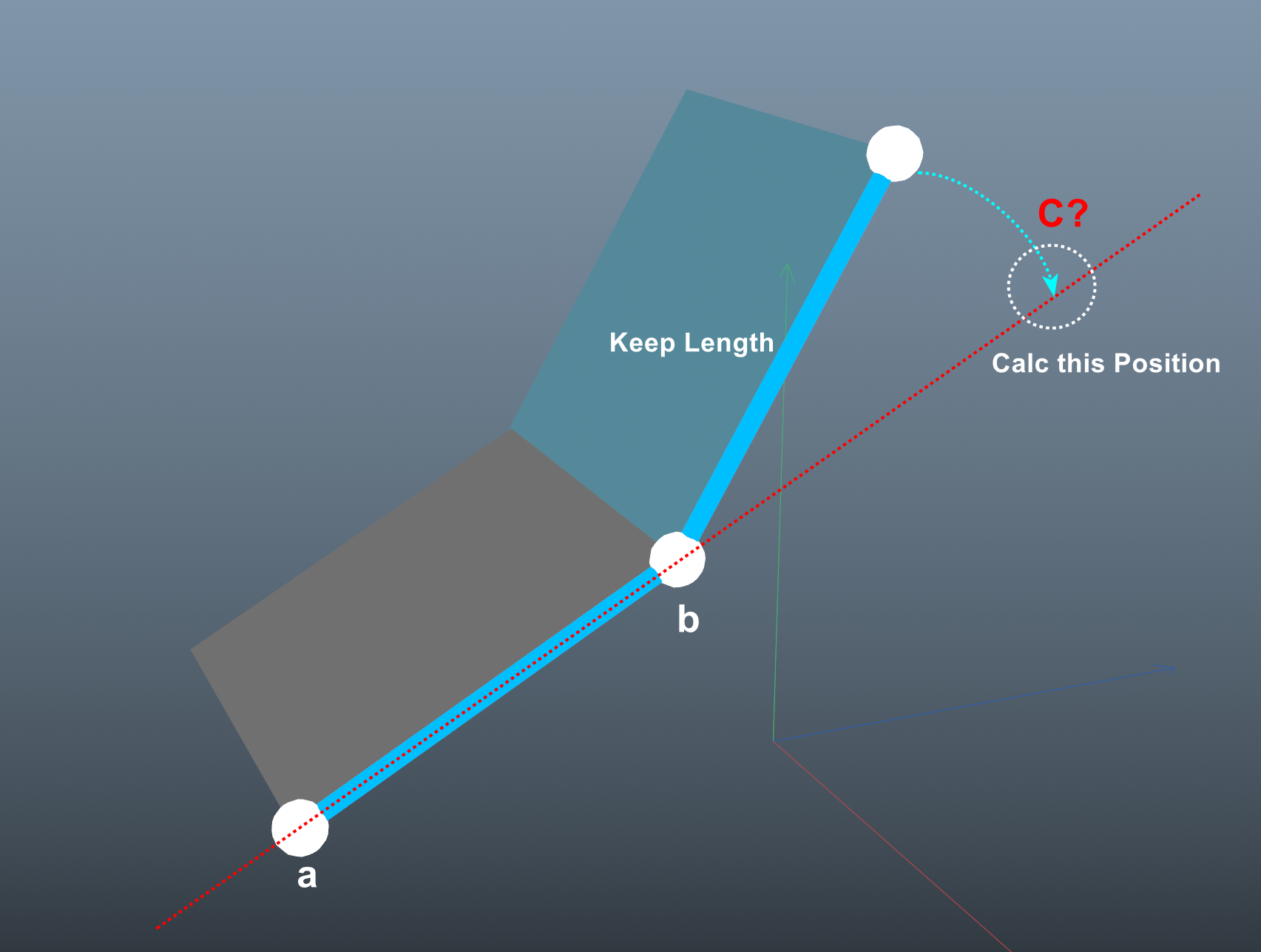# Solved Calculate third point position aligned with two points(edge dir)

There are three points. In the picture, I want to straighten the third point while keeping the edge length. What are the correct methods for vector and matrix arithmetic (Python/R2023)? Thank You.

test.c4dHere is one of the calculations：
In world coordinates, get ab vector and the length of bc vector
Then normalize the ab vector , then use the length of the bc vector to scale the length of the has normalized ab vector , use point b world position to offset the ab vector (that is, add the world position of the point b vector) to get the position of point C

file use Xpresso to calculate C Position
test.c4d

Here is one of the calculations：
In world coordinates, get ab vector and the length of bc vector
Then normalize the ab vector , then use the length of the bc vector to scale the length of the has normalized ab vector , use point b world position to offset the ab vector (that is, add the world position of the point b vector) to get the position of point C

file use Xpresso to calculate C Position
test.c4d

How do I change this to run in the local coordinate system?
I'm not familiar with the matrix. Thank you.

ah... sorry forget it. remove * mg

Hi @ymoon,